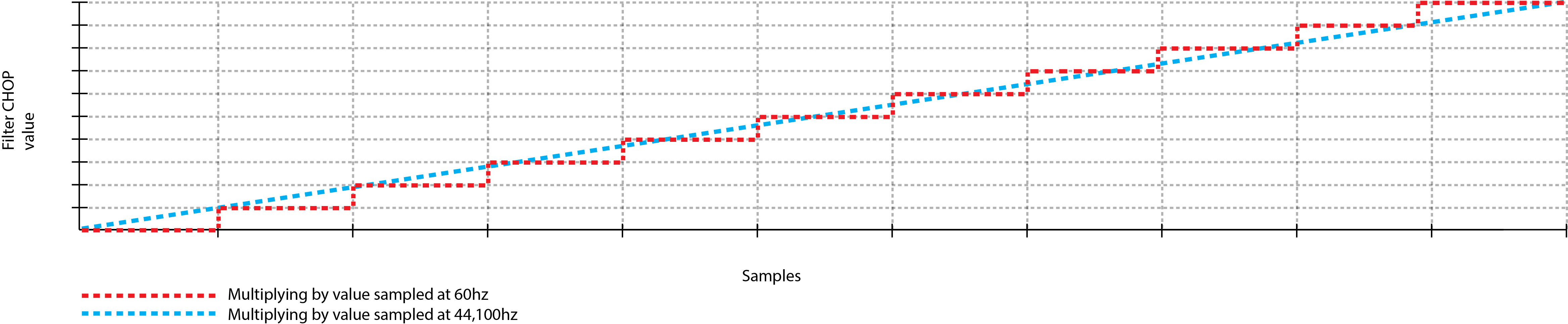### 4.4 Sample Rates

Many applications never expose the granular functions and operations that are happening behind the scenes. Because of this, many people aren't used to processing audio in a mathematical fashion. Audio is, at its core, numerical data being processed incredibly fast. Knowing this lays the groundwork for how to work with audio in TouchDesigner.

Open up example 'Sample_rates_1.toe'. This example creates a very basic feature common in many audio applications: muting. This is achieved by using a Math CHOP to multiply the audio stream by the output value of a Button COMP, which is either 0 or 1. Like any other mathematical equation, a value, in this case each sample of audio, multiplied by 0 will always be 0. Similarly, a value, or audio sample, multiplied by 1 will be returned unchanged. These two states produce on and off states for this audio example.

Let's take this example a step further by allowing the button to fade the audio in and out. Open example 'Sample_rates_2.toe'.

This example takes the previous example and adds two Operators. The first is the Filter CHOP which smoothens the input value. This creates a smooth ramp between the two states of the button. The second is a Resample CHOP.

The sample rate of different Operators is something that is overlooked by many beginners, but is essential to having artifact-free audio. The Oscillator CHOP is being sampled 44,100 times a second, and the Filter CHOP is being sampled 60 times a second. This discrepancy means that there will not be a 1:1 ratio between the samples of audio and the samples of the ramp when they are multiplied. More accurately, there will be a 735:1 ratio between samples of audio and samples of the ramp. This means when the two values are multiplied, the audio will step up or down in volume every 735 samples. Examine the diagram below, where the dotted blue line is a 1:1 ratio, and the dotted red line represents a 735:1 ratio.Looking at the diagram above, there is a very distinct stepping that happens when the two channels that have different sample rates are multiplied. Many CHOPs use the project FPS as their default sample rate, causing the stepping to become exaggerated when the project is set to run at 30 FPS. Using the same example as above, the ratio of samples of audio and samples of the ramp would jump from 735:1 to 1470:1. This means in a 30 FPS project, there would only be an incremental volume change every 1470 samples!

The above examples highlight the need to always be aware of the sample rates of CHOPs in a project, and to use the Resample CHOP when necessary. Many times, this situation will occur in regards to audio, but there are instances where control data might need to be input or output at a different sample rate.